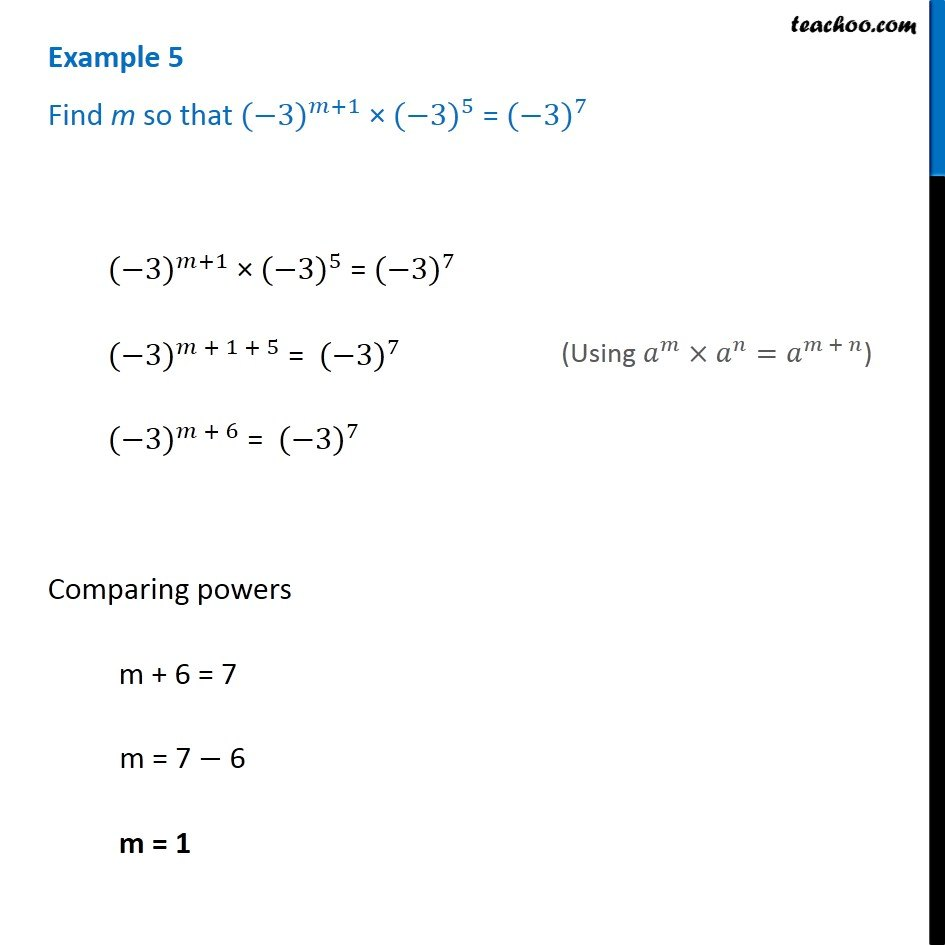1. Chapter 12 Class 8 Exponents and Powers
2. Serial order wise
3. Examples

Transcript

Example 5 Find m so that 〖(−3)〗^(𝑚+1) × 〖(−3)〗^5 = 〖(−3)〗^7 〖(−3)〗^(𝑚+1) × 〖(−3)〗^5 = 〖(−3)〗^7 〖(−3)〗^(𝑚 + 1 + 5) = 〖(−3)〗^7 〖(−3)〗^(𝑚 + 6) = 〖(−3)〗^7 Comparing powers m + 6 = 7 m = 7 − 6 m = 1 (Using 𝑎^𝑚×𝑎^𝑛=𝑎^(𝑚 + 𝑛))

Examples

About the AuthorDavneet Singh
Davneet Singh is a graduate from Indian Institute of Technology, Kanpur. He has been teaching from the past 10 years. He provides courses for Maths and Science at Teachoo.